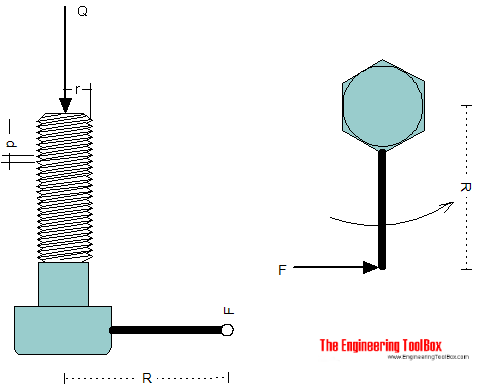Engineering ToolBox - Resources, Tools and Basic Information for Engineering and Design of Technical Applications!

# Screw Jack - Effort Force vs. Load

## Screw jacks and effort forces.

A screw jack is a mechanical device that can increase the magnitude of an effort force.The effort force for a screw jack when neglecting friction can be expressed as

F = (Q p) / (2 π R)                                 (1)

where

F = effort force at the end of the arm or handle (lb, N)

Q = weight or load (lb, N)

p = pitch - distance or lead of thread in one turn  (in, m)

R = lever-arm radius (in, m)

The torque acting on the screw can be calculated as

T = F R                                 (2)

where

T = torque (lb in, Nm)

### Example - Screw jack without friction

If the load on a screw jack is 50000 lb, the lever-arm is 20 inches and the pitch equals 1/8 inches - the effort force can be estimated to

F = ((50000 lb) (1/8 in)) / (2 π (20 in))

= 49.8 lb

The torque acting on the screw can be calculated

T = (49.8 lb) (20 in)

= 995 lb in

= 82.9 lb ft

### Example - Metric Screw Lift

A M24 metric screw with pitch 3 mm is used in a mechanical screw lift with load 225 kN. The lever arm is 0.5 m. The effort force required can be calculated as

F = ((225000 N) (0.003 m)) / (2 π (0.5 m))

= 215 N

The torque acting on the screw can be calculated

T = (215 N) (0.5 m)

= 107 Nm

### Screw Jack Calculator - without Friction - Imperial Units

Calculate torque and effort force acting on the lever arm.

lever arm (in)

### Screw Jack Calculator - without Friction - Metric Units

Calculate torque and effort force acting on the lever arm.

lever arm (m)

### Screw Jack with Friction

For motion in the same direction as the load (load assist the screw jack), the effort force can expressed as

F = Q ( (2 π μ r - p) / (2 π r + μ p) ) (r / R)                                   (2)

where

r = pitch radius of screw (in)

μ = friction coefficient

For motion in the opposite direction as the load (load resists the screw jack), the effort force can expressed as

F = Q ( (2 π μ r + p) / (2 π r - μ p) ) (r / R)                                 (3)

## Related Topics

• Mechanics - Forces, acceleration, displacement, vectors, motion, momentum, energy of objects and more.
• Statics - Loads - forces and torque, beams and columns.

## Engineering ToolBox - SketchUp Extension - Online 3D modeling!

Add standard and customized parametric components - like flange beams, lumbers, piping, stairs and more - to your Sketchup model with the Engineering ToolBox - SketchUp Extension - enabled for use with the amazing, fun and free SketchUp Make and SketchUp Pro .Add the Engineering ToolBox extension to your SketchUp from the SketchUp Pro Sketchup Extension Warehouse!

Translate

## Privacy

We don't collect information from our users. Only emails and answers are saved in our archive. Cookies are only used in the browser to improve user experience.

Some of our calculators and applications let you save application data to your local computer. These applications will - due to browser restrictions - send data between your browser and our server. We don't save this data.

## Citation

• Engineering ToolBox, (2009). Screw Jack - Effort Force vs. Load. [online] Available at: https://www.engineeringtoolbox.com/screw-jack-d_1308.html [Accessed Day Mo. Year].

Modify access date.

. .

#### Scientific Online Calculator3 30